# Test: Laminar Flow in Pipes - 2

## 6 Questions MCQ Test Topicwise Question Bank for Civil Engineering | Test: Laminar Flow in Pipes - 2

Description
Attempt Test: Laminar Flow in Pipes - 2 | 6 questions in 15 minutes | Mock test for Civil Engineering (CE) preparation | Free important questions MCQ to study Topicwise Question Bank for Civil Engineering for Civil Engineering (CE) Exam | Download free PDF with solutions
QUESTION: 1

### A fluid of kinematic viscosity 0.4 cm2/sec flows through a 8 cm diameter pipe. The maximum velocity for laminar flow will be

Solution:

Maximum Reynold's number for laminar flow = 2000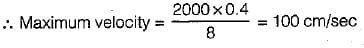QUESTION: 2

Solution:
QUESTION: 3

### In a two-dimensional flow of a viscous fluid couette flow is defined for

Solution:

Coutte flow is characterized as flow of very low value of Reynolds number between two parallel plate, one is fixed and other is movable.

QUESTION: 4

Consider the following statements regarding the laminar flow through a circular pipe:
1. The friction factor is constant.
2. Thp friction factor dspends Upon the pipe roughness.
3. The friction factor varies inversely with the Reynolds number of flow.
4. The velocity distribution is parabolic.
5. The pressure drop varies directly with the mean velocity.
Of these statements:

Solution:

For laminar flow th rough circular pipe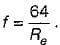It is neither constant, and does not depend on pipe roughness.
As head loss varies directly with mean velocity so pressure drop also.

QUESTION: 5

The velocity profile in fully developed laminar flow in a pipe of diameter D is given by u = u0(1 - 4r2/D2), where r is the radial distance from the center. If the viscosity of the fluid is μ, the pressure drop across a length L of the pipe is

Solution:

We Know that,
Pressure drop across a length L of pipe is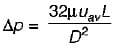...(i)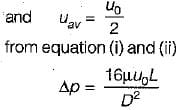QUESTION: 6

Laminar developed flow at an average velocity of 5 m/s occurs in a pipe of 10 cm radius. The velocity at 5 cm radius is

Solution: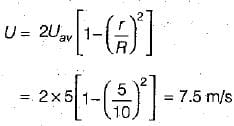Use Code STAYHOME200 and get INR 200 additional OFF Use Coupon Code